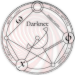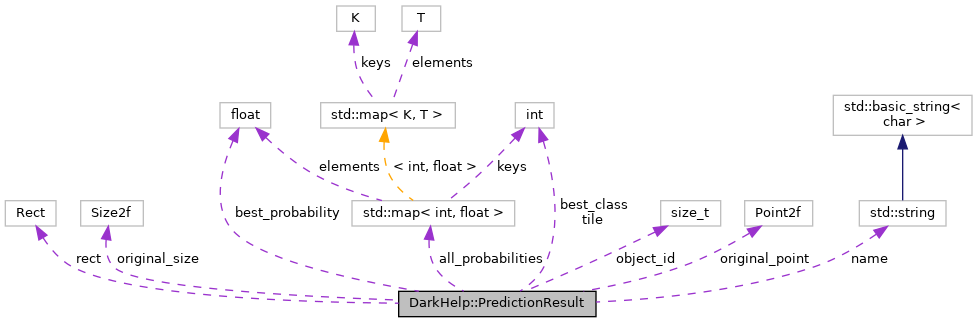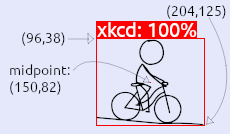DarkHelp  v1.1.16-1 C++ API for the neural network framework Darknet Looking for a C++ dev who knows OpenCV?I'm looking for work. Hire me!DarkHelp::PredictionResult Struct Reference

Structure used to store interesting information on predictions. More...

Collaboration diagram for DarkHelp::PredictionResult:[legend]

## Public Attributes

cv::Rect rect
OpenCV rectangle which describes where the object is located in the original image. More...

cv::Point2f original_point
The original normalized X and Y coordinate returned by darknet. More...

cv::Size2f original_size
The original normalized width and height returned by darknet. More...

MClassProbabilities all_probabilities
This is only useful if you have multiple classes, and an object may be one of several possible classes. More...

int best_class
The class that obtained the highest probability. More...

float best_probability
The probability of the class that obtained the highest value. More...

std::string name
A name to use for the object. More...

int tile
The tile number on which this object was found. More...

## Detailed Description

Structure used to store interesting information on predictions.

A vector of these is created and returned to the caller every time predict() is called. The most recent predictions are also stored in prediction_results.

## ◆ rect

 cv::Rect DarkHelp::PredictionResult::rect

OpenCV rectangle which describes where the object is located in the original image.

Given this example annotated 230x134 image:The red rectangle returned would be:

• `rect.x` = 96 (top left)
• `rect.y` = 38 (top left)
• `rect.width` = 108
• `rect.height` = 87
original_point
original_size

## ◆ original_point

 cv::Point2f DarkHelp::PredictionResult::original_point

The original normalized X and Y coordinate returned by darknet.

This is the normalized mid-point, not the corner. If in doubt, you probably want to use `rect.x` and `rect.y` instead of this value.

Given this example annotated 230x134 image:The `original_point` returned would be:

• `original_point.x` = 0.652174 (mid x / image width, or 150 / 230)
• `original_point.y` = 0.608209 (mid y / image height, or 81.5 / 134)
rect
original_size

## ◆ original_size

 cv::Size2f DarkHelp::PredictionResult::original_size

The original normalized width and height returned by darknet.

If in doubt, you probably want to use `rect.width` and `rect.height` instead of this value.

Given this example annotated 230x134 image:The `original_size` returned would be:

• `original_size.width` = 0.469565 (rect width / image width, or 108 / 230)
• `original_size.height` = 0.649254 (rect height / image height, or 87 / 134)
rect
original_point

## ◆ all_probabilities

 MClassProbabilities DarkHelp::PredictionResult::all_probabilities

This is only useful if you have multiple classes, and an object may be one of several possible classes.

Note
This will contain all non-zero class/probability pairs.

For example, if your classes in your `names` file are defined like this:

car
person
truck
bus

Then an image of a truck may be 10.5% car, 0% person, 95.8% truck, and 60.3% bus. Only the non-zero values are ever stored in this map, which for this example would be the following:

• 0 -> 0.105 // car
• 2 -> 0.958 // truck
• 3 -> 0.603 // bus

The C++ map would contains the following values:

all_probabilities = { {0, 0.105}, {2, 0.958}, {3, 0.603} };

(Note how `person` is not stored in the map, since the probability for that class is 0%.)

In addition to `all_probabilities`, the best results will also be duplicated in best_class and best_probability, which in this example would contain the values representing the truck:

## ◆ best_class

 int DarkHelp::PredictionResult::best_class

The class that obtained the highest probability.

For example, if an object is predicted to be 80% car or 60% truck, then the class id of the car would be stored in this variable.

best_probability
all_probabilities

## ◆ best_probability

 float DarkHelp::PredictionResult::best_probability

The probability of the class that obtained the highest value.

For example, if an object is predicted to be 80% car or 60% truck, then the value of 0.80 would be stored in this variable.

best_class
all_probabilities

## ◆ name

 std::string DarkHelp::PredictionResult::name

A name to use for the object.

If an object has multiple probabilities, then the one with the highest probability will be listed first. For example, a name could be `"car 80%, truck 60%"`. The `name` is used as a label when calling annotate().

names_include_percentage

## ◆ tile

 int DarkHelp::PredictionResult::tile

The tile number on which this object was found.

This is mostly for debug purposes and only tiling has been enabled (see DarkHelp::enable_tiles), otherwise the value will always be zero.

The documentation for this struct was generated from the following file:
• DarkHelp.hpp
DarkHelp::PredictionResult::all_probabilities
MClassProbabilities all_probabilities
This is only useful if you have multiple classes, and an object may be one of several possible classe...
Definition: DarkHelp.hpp:170